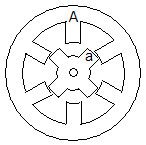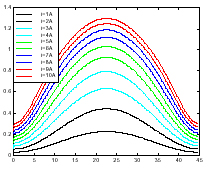Basic of SR Motor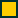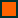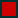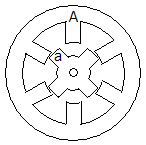What are the Characteristics of a Switched Reluctance Motor?

The flux linkage, inductance and torque characteristics of a SR motor vary with the rotor position (i.e. the relative position of the rotor pole with the stator pole). The characteristics are in cyclic nature and repeated at every rotor pole pitch. Figure 4a shows the rotor pole 'a' is unaligned with stator pole 'A'. Figure 4b shows the same rotor pole in a new unaligned position with the stator pole 'A' after a rotation of 90 degree in clockwise direction. The angle between these two positions is defined as the rotor pole pitch.

Typical flux linkage, inductance and torque characteristic of a SR motor are shown in Figure 5a, Figure 5b and Figure 5c. The characteristics represent a 12/8 3-phase SR motor which has a rotor pole pitch of 45 degree. A 12/8 3-phase SR motor is effectively a 6/4 3-phase SR motor with a 'multiplicity' of two. In Figure 5c, only positive torque is shown and it is plotted over half a rotor pole pitch of 22.5 degree. It can seen that the flux linkage, inductance and torque characteristics of a SR motor are highly non-linear.

Figure 4a
Figure 4b
Figure 5a
Figure 5b
Figure 5c